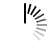# C++ Program to Find Fibonacci Numbers using Matrix Exponentiation

The Fibonacci numbers, commonly denoted Fn form a sequence, called the Fibonacci sequence, i.e; each number is the sum of the two preceding ones, starting from 0 and 1. That is −

```F0 = 0 and F1 = 1
And
Fn = Fn-1 + Fn-2
for n > 1.```

## Algorithm

```Begin
Take two 2 dimensional array
Create a function and Perform matrix multiplication
Create another function to find out power of matrix
Create a function then to find out the Fibonacci number
Multiply(arr1, arr2)
Take 4 variables a, b, c, d
a = arr1 * arr2 + arr1 * arr2
b= arr1 * arr2 + arr1 * arr2
c = arr1 * arr2 + arr1 * arr2
d = arr1 * arr2 + arr1 * arr2
arr1 = a
arr1 = b
arr1 = c
arr1 = d
Power(arr1, take integer n as input)
if (n == 0 or n == 1)
return;
arr1  = {{1,1}, {1,0}}
power(arr1, n / 2)
multiply(arr1, arr1)
if (n mod 2 not equal to 0)
multiply(arr1, arr2)
fibonacci_matrix(n)
arr1 = {{1,1}, {1,0}}
if n ==0
return 0
power(arr1 n - 1)
return arr1
End```

## Example Code

```#include <iostream>
using namespace std;
void multiply(int F, int M) {
int a = F * M + F * M;
int b= F * M + F * M;
int c = F * M + F * M;
int d = F * M + F * M;
F = a;
F = b;
F = c;
F = d;
}
void power(int F, int n) {
if (n == 0 || n == 1)
return;
int M = {{1,1},{1,0}};
power(F, n / 2);
multiply(F, F);
if (n % 2 != 0)
multiply(F, M);
}
int fibonacci_matrix(int n) {
int F = {{1,1},{1,0}};
if (n == 0)
return 0;
power(F, n - 1);
return F;
}
int main() {
int n;
while (1) {
cout<<"Enter the integer n to find nth fibonacci no. (enter 0 to exit):";
cin>>n;
if (n == 0)
break;
cout<<fibonacci_matrix(n)<<endl;
}
return 0;
}```

## Output

```Enter the integer n to find nth fibonacci no. (enter 0 to exit): 2
1
Enter the integer n to find nth fibonacci no. (enter 0 to exit): 6
8
Enter the integer n to find nth fibonacci no. (enter 0 to exit): 7
13
Enter the integer n to find nth fibonacci no. (enter 0 to exit): 0```